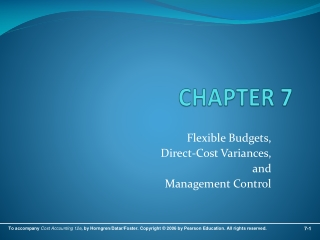DownloadDownload PresentationCHAPTER 7

# CHAPTER 7

Télécharger la présentation## CHAPTER 7

- - - - - - - - - - - - - - - - - - - - - - - - - - - E N D - - - - - - - - - - - - - - - - - - - - - - - - - - -
##### Presentation Transcript

1. CHAPTER 7 Flexible Budgets, Direct-Cost Variances, and Management Control

2. Basic Concepts • Variance – difference between an actual and an expected (budgeted) amount • Management by Exception – the practice of focusing attention on areas not operating as expected (budgeted) • Static (Master) Budget – is based on the output planned at the start of the budget period

3. Basic Concepts • Static-Budget Variance (Level 0) – the difference between the actual result and the corresponding static budget amount • Favorable Variance (F) – has the effect of increasing operating income relative to the budget amount • Unfavorable Variance (U) – has the effect of decreasing operating income relative to the budget amount

4. Variances • Variances may start out “at the top” with a Level 0 analysis • This is the highest level of analysis, a super-macro view of operating results • The Level 0 analysis is nothing more than the difference between actual and static-budget operating income

5. Variances • Further analysis decomposes (breaks down) the Level 0 analysis into progressively smaller and smaller components • Answers: “How much were we off?” • Levels 1, 2, and 3 examine the Level 0 variance into progressively more-detailed levels of analysis • Answers: “Where and why were we off?”

6. A Simple Example • Operating Indicators:

7. A Simple Example Level 1 Analysis Level 0 Analysis

8. Evaluation • Level 0 tells the user very little other than how much Contribution Margin was off from budget: a \$680 F variance in this case • Level 0 answers the question: “How much were we off in total?” • Level 1 gives the user a little more information: it shows which line-items led to the total Level 0 variance • Level 1 answers the question: “Where were we off?”

9. Flexible Budget • Flexible Budget – shifts budgeted revenues and costs up and down based on actual operating results (activities) • Represents a blending of actual activities and budgeted dollar amounts • Will allow for preparation of Levels 2 and 3 variances • Answers the question: “Why were we off?”

10. A Flexible-Budget Example Level 3 Variances will explore these figures in detail

11. Level 3 Variances • All Product Costs can have Level 3 Variances. Direct Materials and Direct Labor will be handled next. Overhead Variances are discussed in detail in a later chapter • Both Direct Materials and Direct Labor have both Price and Efficiency Variances, and their formulae are the same

12. Level 3 Variances • Price Variance formula: • Efficiency Variance formula:

13. Variances and Journal Entries • Each variance may be journalized • Each variance has its own account • Favorable variances are credits; Unfavorable variances are debits • Variance accounts are generally closed into Cost of Goods Sold at the end of the period, if immaterial

14. Standard Costing • Budgeted amounts and rates are actually booked into the accounting system • These budgeted amounts contrast with actual activity and give rise to Variance accounts

15. Standard Costing • Reasons for implementation: • Improved software systems • Wide usefulness of variance information

16. Management Uses of Variances • To understand underlying causes of variances • Recognition of inter-relatedness of variances • Performance Measurement • Managers ability to be Effective • Managers ability to be Efficient

17. Activity-Based Costing and Variances • ABC easily lends itself to budgeting and variance analysis • Budgeting is not conducted on the departmental-wide basis (or other macro approaches) • Instead, budgets are built from the bottom-up with activities serving as the building blocks of the process